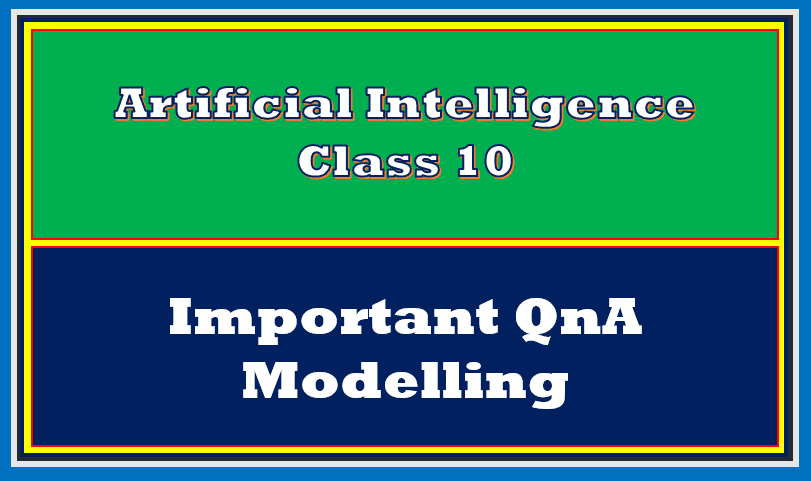In this article we are going to discuss important QnA modelling AI class 10. So here we go!

Topics Covered

## Important QnA Modelling AI Class 10

Let us begin the article important QnA Modelling AI class 10, with Objective Type Questions including fill in the blanks, true and false and MCQs.

## Objective Type Questions (OTQs)

1. The ________ methods helps to use data for making predictions or future forecasts. (Ans. modelling)
2. Which of the following not an approach considered for modelling:
• rule-based approach
• learning-based approach
• knowledge-based approch
• All of these
3. The ________ approach is based on rules and data fed into the machine. (Ans. rule-based)
4. In rule-based apporach the relationships or patterns in data are defined by the developer. (True/False)
5. The ___________ is used to make a successful model using rule-based approach. (Ans. coding)
6. In _________ approach of the modelling, machine designs its own algorithm for data. (Ans. learning-based)
7. In learning-based approach, data can be taken randomly from anywhere. (True/False)
8. The ___________ approach can be used when data is labelled. (Ans. rule-based)
9. The __________ follows tree like structure of the decisions with all possible results. (Ans. decision tree)
10. The top most node of decision tree is known as ___________ (Ans. root)
11. Which of the following node is the last node of a decision tree?
• root
• terminal
• interior
• parent
12. ________ is a process by which a node is divided into two or more sub-nodes. (Ans. Splitting)

In the next section of important QnA Modelling AI Class 10, I am going to discuss subjective type questions.

## Subjective Type Questions (STQs)

Let us see the STQs for Modelling AI Class 10.

1. What are the main approaches used for AI modelling?
• There are two approaches mainly used for AI modelling:
• Rule-Based Approach
• Learning-Based Approach
2. Explain rule-based approach in detail.
• A Rule-based approach is generally based on the data and rules fed to the machine, where the machine reacts accordingly to deliver the desired output.
• It follows the relationship or patterns in data defined by the developer.
• The machine follows the instructions or rules mentioned by the developer and performs the tasks accordingly.
• It uses coding to make a successful model.
3. Explain learning-based approach in detail.
• The machine is fed with data and the desired output to which the machine designs its own algorithm (or set of rules) to match the data to the desired output fed into the machine to train.
• In the learning-based approach, the relationship or pattern in data is not defined by the developer.
• This approach takes random data which is fed into the machine and it is left to the machine to figure out the patterns or required trends.
• In general this approach is useful when the data is not labelled and random for a human to use them.
• Thus, the machine looks at the data, tries to extract similar features out of it and clusters the same datasets together.
• In the end as output, the machine tells us about the trends which are observed in the training data.
• This approach is used to train the data which is unpredictable or the users have no idea about it.
4. What do you mean by decision tree?
• A decision tree is a very useful for modelling in business.
• It follows a tree like structure of decisions with all possible outputs.
• The top most node of decision tree is known as root node.
• Every node is connected with lines.
• It follows top-bottom approach. The root node is always on top and the terminal node is at the bottom.
5. Define the followiing terms:
• Root Node: The top node of decision tree is known as root node.
• Splitting: Splitting is a process by which a node is divided into two or more sub-nodes.
• Decision or interior node: It is the node where the splitting takes place. In other words, it is a place where the sub-node is divided into another sub-nodes.
• Leaf node or terminal node: The bottom node is known as leaf node or terminal node.
• Branch or Subtree: A subsection of the decision tree is known as a branch or subtree.
• Parent node and child node: The bottom node which derives from the top node is known as child node whereas the top node is known as the parent node.

That’s all from important QnA Modelling AI Class 10. If you have any doubt or query related to this article Modelling AI Class 10, feel free to ask in the comment section.